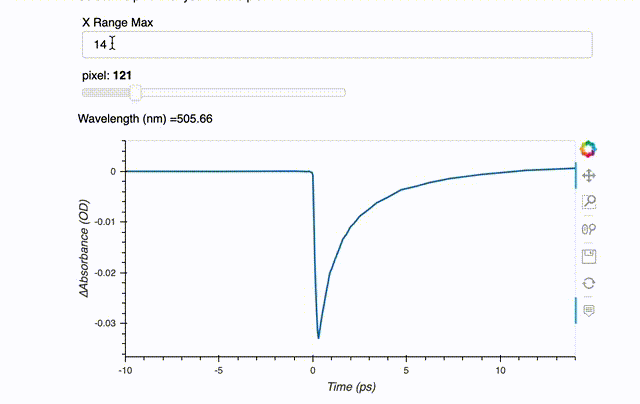# How to implement Range Slider For X and Y axis in the Figure?

Hello,

I am trying to implement range slider into my figure. I basically want to have a range-slider (with two values) to use for x/y axis range definition in the figures. At the moment, I have one slider (not a range) to define maximum value of X-axis range in the figure. However, it does not work in real-time. Somehow, I have to callback my function to re-plot the figure whenever the value of slider changes. I checked a lot of documentation but could not find a satisfactory answer.This is my code below. Any guidence, help is highly appreciated!

``````import pandas as pd
import numpy as np
import panel as pn
import matplotlib.pyplot as plt
import hvplot.pandas
from matplotlib.figure import Figure
from matplotlib.backends.backend_agg import FigureCanvas
from decimal import Decimal

pn.extension()

%matplotlib inline
%matplotlib notebook

# Global variables
fig_width = 600
fig_height = 300

d = np.genfromtxt('visdata.dat', delimiter='\t')
row_data = pd.DataFrame(data=d[0:,0:])
d1 = row_data.iloc[1:,0:] # we use this data frame for plotting time traces.
tim_values = row_data.iloc[1:,0]
min_tim = int(tim_values.min())
max_tim = int(tim_values.max())

x_range_max = pn.widgets.Spinner(name='X Range Max', value=5, step=1, start=min_tim, end=max_tim)

def plot_timtrace(pixel = 1):
fig1 = d1.hvplot.line(x='0',
y=f'{pixel}',
xlim=(-10, x_range_max.value),
xlabel='Time (ps)',
ylabel='∆Absorbance (OD)',
width = fig_width,
height = fig_height)
return pn.Column(f'Wavelength (nm) ={row_data.iloc[0,pixel]}',
fig1.opts(axiswise=True))

wavelen_text = pn.interact(plot_timtrace,
pixel=range(1,len(d1.columns)))

graph1 = pn.Column("<br>\n#Time-Traces\nSelect the pixel that you want to plot.",x_range_max,wavelen_text)``````

Hi, if I understand correctly, you want to change in real-time the range of your X and Y-axis thanks to two range sliders.

Maybe this is what you’re looking for :

``````import param
import panel as pn
import holoviews as hv

class AppTest(param.Parameterized):
rangeX_ = param.Range(default=(-10,20),bounds=(-50,50))
rangeY_ = param.Range(default=(-10,20),bounds=(-50,50))
plot = hv.Curve[(0,0),(20,20)])

@param.depends('rangeX_',rangeY_')
def view(self):
return self.plot.redim.range(x=self.rangeX_,y=self.rangeY_)

viewer = AppTest()
pn.extension()
pn.Row(pn.Param(viewer.param),viewer.view)``````